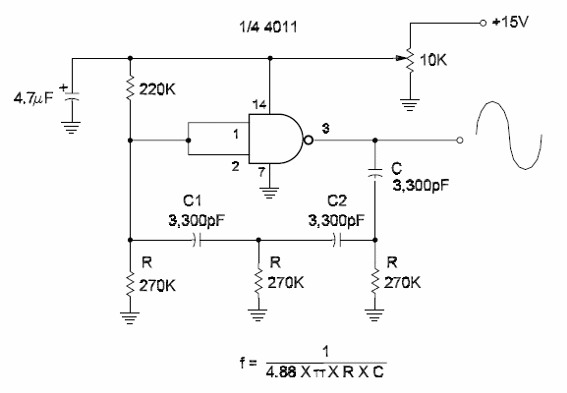### DatasheetsN° of component

## Sine Wave Oscillator Using an lnverter ll (CIR098E)

Figure bellow shows another configuratíon for a Iow-frequency sine wave oscillator using CMOS Iogic. One inverter of common CMOS functions, such as the 4001 or 4011 can be used in this phase-shift oscillaton The frequency depends on the capacitors in the RC network and the resistors. The trimmer potentiometer is adjusted to the point where the voltage supplied to the CMOS block finds the correct point in the Iinear mode of operation. When designing a circuit for any frequency, keep the resistors with the values as recommended in this block and calculate the capacitors. This circuit is suitable for audio signals in the band between a Iow hertz and 10 kHz. When calculating the components for a new frequency, keep the resistors with the values as suggested and calculate C.Sine Wave Oscillator Using an lnverter ll